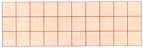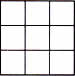Friday , August 19 2022# NCERT 5th Class (CBSE) Mathematics: Area And Volume

### A. Area of

Mrs Rao has recently put new tiles in her kitchen. One way to find the are covered by the tiles would be to count them. The other way would be to use multiplication.There are 10 tiles in a row. There are 3 rows of tiles

10 × 3 = 30 tiles

Area = 30 sq. units

This is the same as multiplying the length and breadth of the rectangle.

10       ×          3      =     30 sq. units

Length × breadth = Area of a rectangle ← Formula for areas of a rectengle

(a) Length = 20 cm, Breadth = 0.1 m, Area = ?

0.1 m = 10 cm

20 cm × 10 cm = 200 sq. cm

Area = 200 cm2

(b) Area of a rectangle = 72 m2

Length = 12 m

72/12 = 6

### B. Area of a Square

A square is a rectangle with all sides equal.

So, the area of a square = side × Side ← Formula for areas of a square

(a)Side of the square = 3 units

Area = 3 × 3 = 9 sq. units

(b)Side of the square = 2 cm

Area =2 × 2 = 4 cm2

## चुनौती हिमालय की 5th NCERT CBSE Hindi Rimjhim Ch 18

चुनौती हिमालय की 5th Class NCERT CBSE Hindi Book Rimjhim Chapter 18 प्रश्न: लद्दाख जम्मू-कश्मीर राज्य में …